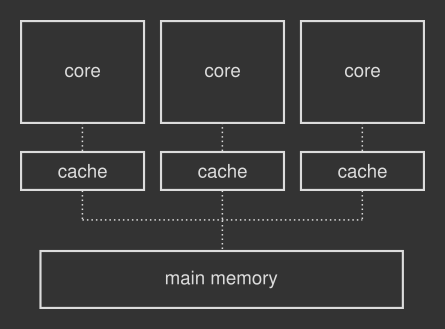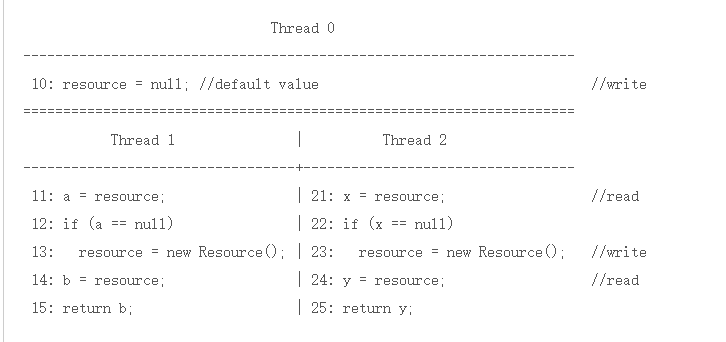# 何为内存重排序？

## 何为重排序`class ReadWriteDemo {      int A = 0;      boolean B = false;        //CPU1 (thread1) runs this method      void writer() {          A = 10;          B = true;      }        //CPU2 (thread2) runs this method      void reader() {          while (!B)              continue;          System.out.println(A == 10);      }  }`

### 指令调度说明

`public class Main {      public static void main(String[] args) {            int A = 10;          int B = A + 10;          int C = 20;      }  }`

`public class UnsafeLazyInitialization {      private static Resource resource;        public static Resource getInstance() {          if (resource == null)              resource = new Resource();          return resource;      }  }``public class UnsafeLazyInitialization {      private static Resource resource;        public static Resource getInstance() {          Resource temp = resource;          if (resource == null)              resource = temp = new Resource();          return temp;      }  }`